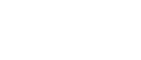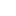#• 产品• 解决方案• 技术• 支持• 关于我们中国 | 中文• Japan
• Global
• United States
• Europe
• China
• Korea
• Taiwan
• India

#• 产品
• 解决方案
• 技术
• 支持
• 关于我们

# 高分辨率解码器元件 (半导体磁阻元件)

## 与m=0.8的齿轮组合使用 差分信号输出方式SMRE

MS0083

MS0083最适用于约Φ60mm～Φ100mm的齿轮，通过区分A+/A-和B+/B-的输出信号，可以降低共模噪声。AKM的SMRE具有极好的温度特性和较小的设备间差异。MS0083通过电插值实现了超过20位的高分辨率旋转角度检测，实现了磁阻元件中的顶级性能*。

* 2018年9月底市场调查结果。

● A+/A-和B+/B-的差分输出。
A相B相运用差分输出来消除共模噪声，并提供了双倍的输出振幅，从而有利于磁编码器的高精度化和高分辨率化。

● 特定齿轮的最佳设计

## 产品系列

`Part Number`
```Part
Status```
```Pitch
[mm]```
```Input / Output
Resistance (MIN.)
[Ω]```
```Input / Output
Resistance (TYP.)
[Ω]```
```Input / Output
Resistance (MAX.)
[Ω]```
`Number of Elements`
`Other`
```Operating
Temperature
[℃]```
```Device
Package```
```Number
of Pins```
`MS0042`
`MP`
`1.28`
`290`

`420`
`2`

`-40 to 125`
`SON`
`8`
`MSP254`
`MP`
`8.64`
`290`

`420`
`4`

`-40 to 125`
`DIP`
`8`
`MSP042`
`MP`
`1.28`
`230`

`375`
`2`

`-40 to 100`
`DIP`
`8`
`MS0082`
`MP`
`2.512`
`465`

`755`
`1`

`-40 to 125`
`SON`
`4`
`MS0030`
`MP`
`0.96`
`270`

`375`
`1`

`-40 to 125`
`SON`
`4`
`MS0043`
`MP`
`1.28`
`275`

`410`
`1`

`-40 to 125`
`SON`
`6`
`MS0050`
`MP`
`1.6`
`270`

`375`
`1`

`-40 to 125`
`SON`
`4`
`MS0020`
`MP`
`0.634`
`205`

`330`
`1`

`-40 to 100`
`SON`
`4`
`MSP204`
`MP`
`6.84`
`290`

`420`
`4`

`-40 to 125`
`DIP`
`8`
`MS0083`
`MP`
`2.624`
`290`

`480`
`1`

`-40 to 125`
`SON`
`6`
`MSP043`
`MP`
`1.28`
`230`

`375`
`2`

`-40 to 100`
`DIP`
`8`
`MS0041`
`MP`
`1.28`
`270`

`375`
`1`

`-40 to 125`
`SON`
`4`
`MSP082`
`MP`
`2.512`
`465`

`755`
`2`

`-40 to 100`
`DIP`
`8`